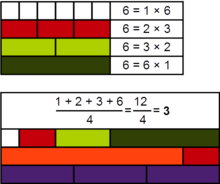# Arithmetic numberDemonstration, with Cuisenaire rods, of the arithmetic nature of the number 6

In number theory, an arithmetic number is an integer for which the average of its positive divisors is also an integer. For instance, 6 is an arithmetic number because the average of its divisors iswhich is also an integer. However, 2 is not an arithmetic number because its only divisors are 1 and 2, and their average 3/2 is not an integer.

The first numbers in the sequence of arithmetic numbers are

1, 3, 5, 6, 7, 11, 13, 14, 15, 17, 19, 20, 21, 22, 23, 27, 29, 30, 31, 33, 35, 37, 38, 39, 41, 42, 43, 44, 45, 46, 47, 49, ... (sequence A003601 in the OEIS).

## Density

It is known that the natural density of such numbers is 1: indeed, the proportion of numbers less than X which are not arithmetic is asymptoticallywhere c = 2 √ log 2 + o(1).

A number N is arithmetic if the number of divisors d(N) divides the sum of divisors σ(N). It is known that the density of integers N obeying the stronger condition that d(N)2 divides σ(N) is 1/2.

## Notes

1. Guy (2004) p.76
2. Bateman, Paul T.; Erdős, Paul; Pomerance, Carl; Straus, E.G. (1981). "The arithmetic mean of the divisors of an integer". In Knopp, M.I. Analytic number theory, Proc. Conf., Temple Univ., 1980 (PDF). Lecture Notes in Mathematics. 899. Springer-Verlag. pp. 197–220. Zbl 0478.10027.